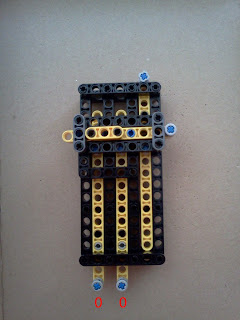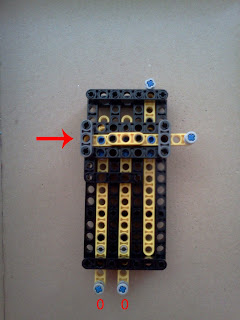Home‎ > ‎Lego Projects‎ > ‎

### Lego Rod Logic

 I've been wanting to create a small "LEGO computer" for a long time. And I've been recently gathering links from related projects, from where I'm taking inspiration (like LEGO Turing machines or the impressive LEGO Difference Engine). While I'm not too impressed with the existing LEGO Turing machines, since they use a computer (the Mindstorms brick) as part of the construction, and thus, they are not purely mechanical machines. The Difference Engine is, however,  by far the most impressive LEGO construction I've ever seen (although it cannot be programmed, and it always performs the same computation). I've been wondering if it's possible to create a purely mechanical LEGO Turing machine. While thinking about how to build it, I found a couple of very interesting pages describing how to mechanically implement Boolean logic using LEGO (both using gears and rods). In particular I think rod logic is very interesting and scalable. You can see a demonstration in this youtube video. So, I decided to try and test some simple logic gates using that technique with LEGO. Here's a small example of an OR gate. But any logic operation can be modeled this way (check out this full adder). The basic idea of rod logic, is to have a series of rods, each of them having a series of pegs in them. Depending on the way you configure the pegs in the rods, some rods might move, or get stuck (thus implementing Boolean logic operations). I'm not going to explain in detail how rod logic works, since that is explained very well in the links above. So, I'll limit myself to explain how does the LEGO rod-logic OR gate works. The gate consists of 4 rods: Input A (left-most vertical rod): This rod can be in two positions, if you push it "up", it means 1, and if you push it "down", it means 0. Input B (middle vertical rod): This rod can be also in the same two positions as A. A temporary rod T (horizontal rod): This rod can be in two positions, if you can push it to the right, then it means 1, if it's stuck on the left, then it means 0. Output C (right most vertical rod): This rod can be in two positions, if you can push it "out", then it means 1, if it's stuck "down", it means 0. As very clearly explained in this page, rod logic is clocked logic. In order to operate the logic gate, we need three time steps: In the initial state, T and C are set to 0, and A and B are set to the desired input values T is pushed right C is pushed up Depending on the values of A and B, when you push T, it might get stuck (0), or it might move (1). The same thing for C. Here you can see 4 sequences of 3 pictures showing the previous 3 time steps for the 4 possible input configurations of AB (00, 01, 10, and 11): Sequence for A = 0, B = 0:In this case, when we push T to the right, there is a peg in T that blocks the movement of C. Thus, when in step 3, we push C up, it does not move. Sequence for A = 0, B = 1: In this case, since B is pushed up, there is a peg in B that blocks the movement of T. Thus, when in step 2, we push T to the right, it does not move. Sequence for A = 1, B = 0: Sequence for A = 1, B = 1: An OR gate is not much, but this is the basic building block for a LEGO computer. Next time, I'll attempt a more complex logical operation. Also, for this to work automatically, I need to build a "clock" mechanism that pushes and pulls the rods at the appropriate time.